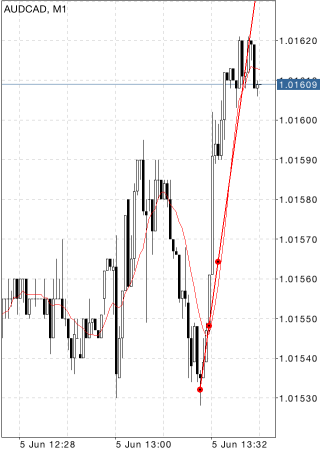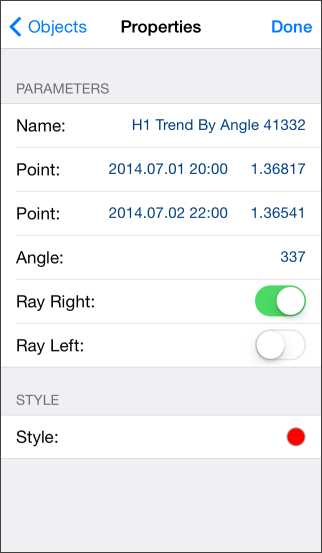# Trendline by Angle

To draw a trendline by angle, select this object, then tap on the chart to choose the initial point of the line and drag your finger to draw a line in the desired direction. Additional parameters will be shown near the end point: distance from the initial point along the time axis, distance from the initial point along the price axis, slope line from the horizontal line drawn through the initial point.## Parameters

There are the following parameters of a trendline by angle:

• Point – coordinates of the initial point (date/value of the price scale);
• Point – coordinates of the end point (date/value of the price scale);
• Angle – slope angle of the trendline from a horizontal line drawn through the initial point;
• Ray Right – infinite duration of a trendline to the right;
• Ray Left – infinite duration of a trendline to the left.

Common parameters of object are described in a separate section.